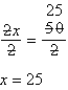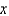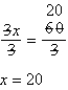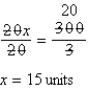# Quiz 2: Ratio and Proportion

Psychology

x = 25 Know Want to Know 2 : 5 :: 10 :Proof: 2 25 = 50 5 10 = 50

x = 20 Know Want to Know 3 : 10 :: 6 :Proof: 3 20 = 60 10 6 = 60

15 units Know Want to Know 20 beds : 1 unit :: 300 beds :unitsProof: 20 15 = 300 1 300 = 300